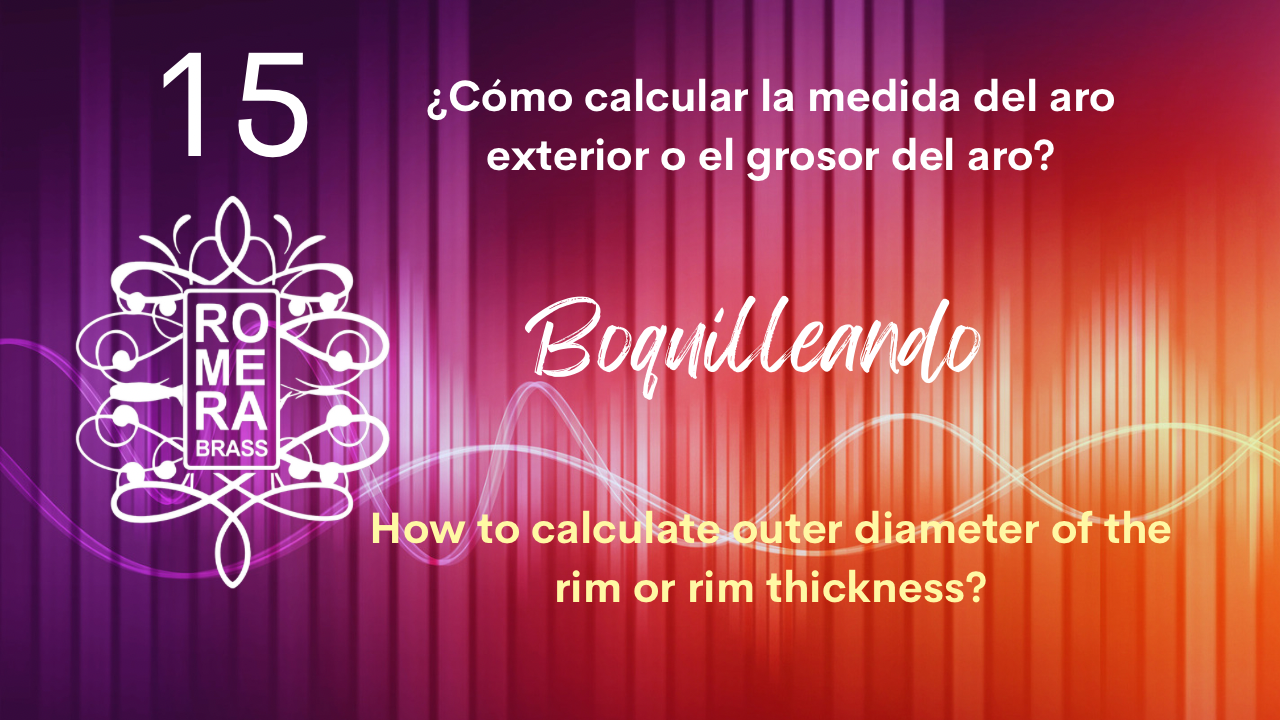# 15. How to calculate outer diameter of the rim or rim thickness?

Today we solve the question that some musicians ask me:

How to calculate outer diameter of the rim or rim thickness? Or… the other way around, how do I know the thickness of the rim if I only have the total diameter of the rim?

Some mouthpieces manufacturers make it easy to measure the thickness of the rim and others, like me, choose to give the outside diameter of the rim.

In this video we are going to see, through very graphic and enlightening examples, how to calculate the measurements of both the thickness of the rim and the outer diameter of the rim. Two very different concepts that should not be confused.

Do not miss it!

Here I leave the formulas!:

Important: To calculate the measurements in both cases we will always need to know the inner diameter of the rim!Greetings and lots of music!

Toni Romera

Below, you will find the transcription of the video to text in case it may be of interest to you to have its content in writing or you want to translate it into another language:

Today we solve the question that some musicians ask me: how to know the total diameter of the hoop if the manufacturer only gives us the thickness of the hoop? or vice versa,

they have the total diameter of the outer rim and want to know the thickness of the rim they are touching.

Some mouthpieces manufacturers give us the thickness of the ring and others, like me on my website, put the total diameter of the outer ring.

I prefer to put this measure since everything is clearer.

In this video we will do some math, but don't worry, it's very basic.

Imagine if they are basic, even I know how to do it! Without further ado, let's begin!

In this video I am going to give just one example of each measurement so as not to make the video too long, and if you want to calculate with other measurements, at the end I will leave you the formulas and you will only have to change the numbers to get the result.

To calculate the measurements in both cases we will always need to know the inside diameter of the ring. I will explain!

We start by calculating the thickness of the hoop. In this case we have a mouthpiece and we have the measurement of the outer diameter of the ring and we want to know the thickness of the ring. We have the total diameter of the rim and we only want to know a part of the thickness of the rim. In this case we need the measurements of the inside diameter of the rim and the outside diameter of the rim.

Let's go with the example:

If we have a mouthpiece with an internal diameter of 17mm and an external diameter of 26mm, the formula is:

The first thing we have to do is take these quantities 26 and 17 and subtract it and the result will be 9 millimeters.

Once we have this measurement we have to divide it by 2 and we will have the result of the thickness of the hoop, in this case 4.5 millimeters.

We will calculate the outside diameter of the hoop if the manufacturer only gives us the thickness of the rim.

Imagine that the manufacturer gives us a thickness of 4.5 millimeters. To calculate the outer diameter we need to know the measurement of the inner diameter. Let's say it has 17 millimeters.

We have to take the measurement of the thickness of the ring of 4.5mm and we add again this quantity 4.5 plus 4.5 the result gives us 9, and now we only have to add the inner diameter of the ring which in this case is 17mm and the result will be 26 millimeters. 26 millimeters is the total measurement of the outside of the rim.

With these simple operations we can already know the total measurements of the outer diameter of the ring or just the thickness of the rim.

I'm Toni Romera and I look forward to your questions and comments.

Greetings and lots of music!